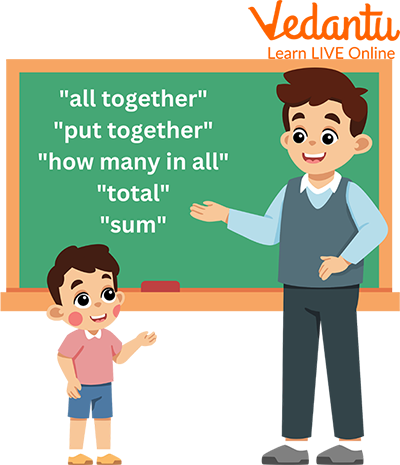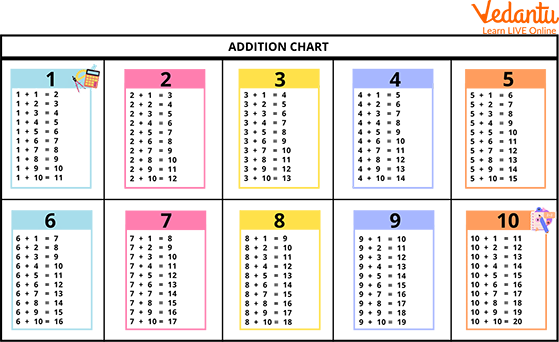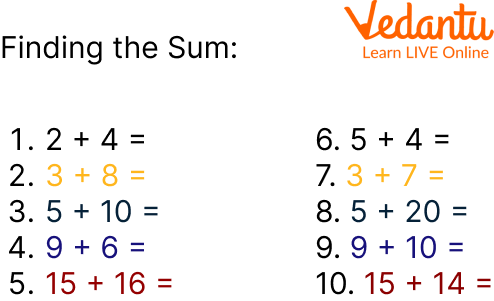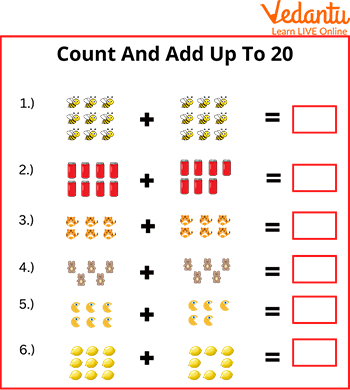Courses
Courses for Kids
Free study material
Free LIVE classes
MoreLIVE
Join Vedantu’s FREE Mastercalss

Addition and subtraction are useful for many activities of everyday life, like setting the table, making changes at the supermarket, and playing some games. The issue with addition that students face is often simply overcome if they follow the idea in a fun way! The game encourages students to count the total numbers. The game implies numbers within 20; students need to be confident in mathematical addition by working at different levels of complexity. In this article, we will learn about finding the sums to 20 and some facts of addition.

## What is a Sum?

Sum is a way of putting things together. The sum brings two or more numbers together to make a new total.Sum

The terms such as "all together, "put together," "how many in all," "total," and "sum" indicate that you will need to add two or more numbers.

The plus sign (+) is used when two things are added together.To find a total of two single-digit numbers, just count the first number on your fingers and then count the second number on your finger, and the sum of total fingers is the result.

For Example: 3 + 3 = 6

1. Counting : Number lines are a great resource for your students. In first grade, labeled and closed number series is a great place to start. Teach your students to start with the largest number and count forward.Counting

2. Zero Facts : Adding zero to something is straightforward and is the same number that is adding to zero.

Any number + zero = the same number (no change)

12 + 0 = 12

0 + 12 = 12

3. Make 10 : As adults, several people automatically create 10s to form friendlier numbers to feature in our heads. Creating ten is such a strategy for our young learners, as well. As soon as addition with regrouping is introduced, problem-solving skills increase significantly. Active creating ten and eventually memorizing that pairs create 10 may be an ability that they're going to fall back on once learning the way to add larger numbers along or once they area unit adding 3+ numbers along

7 + 3 = 10

## Addition Up to 20 Worksheets

Counting sheets with single-digit additions; all sums are less than 20. A horizontal addition format is used. Students can use a variety of problem solving strategies (finger counting, counting, etc.)

##Ans:

1. 2 + 4 = 6

2. 3 + 8 = 11

3. 5 + 10 = 15

4. 9 + 6 = 15

5. 15 + 16 = 31

6. 5 + 4 = 9

7. 3 + 7 = 10

8. 5 + 20 = 25

9. 9 + 10 = 19

10. 15 + 14 = 29Ans:

1. 18

2. 15

3. 12

4. 10

5. 11

6. 17

## Practice QuestionsPractice Question

3 + 1 = 4 3 + 5 = 8 3 + 3 = 6 1 + 6 = 7 4 + 5 =9 6 + 4 = 10

5 + 2 = 7 2 + 3 = 5 7 + 2 = 9 1 + 7 = 8

## Summary

In this article, we got to know about the Addition. Addition and subtraction prepare us for learning about other math topics, including multiplication and division, in school. These addition tricks are a great way to learn mathematics calculations in an easy way for kids. They also help to develop the addition skills and strategies for solving the addition questions and making them more engaging and interesting. And after solving the given worksheets and practice questions, it will be easier for you to find the sum of numbers.

Last updated date: 26th Sep 2023
Total views: 92.1k
Views today: 0.92k

## FAQs on Addition Up to 20

1. Can a 4 year old do addition?

We found children were able to do non-symbolic addition at age 4 and they were able to do symbolic addition at age 5. Children's accuracy of symbolic addition increased greatly after receiving formal school education, and it even exceeded the non-symbolic skills at 7 years old.

2. What's the opposite of sum in math?

The addition means to find the sum, and subtraction means taking away. So, subtraction is the opposite of addition. Hence, addition and subtraction are opposite operations. We may say, subtraction is the inverse operation of addition.

3. Is the sum the same as the total?

Sum and total are amounts. A sum is a total amount through addition. A total is a cumulative amount.